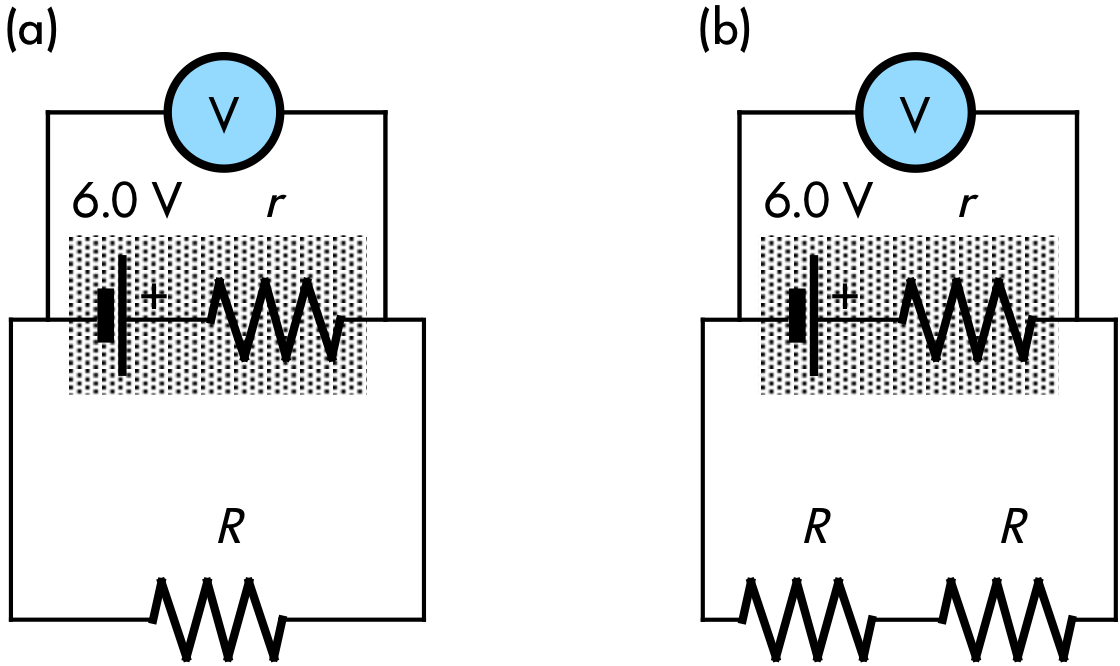## 20180505

### Physics midterm question: changing terminal voltage of real battery

Physics 205B Midterm 2, spring semester 2018
Cuesta College, San Luis Obispo, CAA real battery with an emf of 6.0 V and internal resistance r is connected to a resistor R, and a voltmeter. An additional R resistor is then wired in series to this circuit. Discuss why the voltmeter will have a higher reading for the two-resistor circuit. (Assume that the internal resistance r of the battery remains constant.) Show your work and explain your reasoning using Kirchhoff's rules, Ohm's law, and properties of voltmeters.

• p:
Correct. Discusses/demonstrates how voltmeter in case (b) will have a higher reading because:
1. in both cases, the voltmeter will read the terminal voltage difference across the battery, where the battery's emf ε and internal resistance r are constant:

Ⓥ = +ε − Icircuitr;

2. and in circuit (a) more current will flow compared to circuit (b), as circuit (a) has less equivalent resistance than circuit (b):

1. Icircuit = ΔVeq/Req,

Icircuit = (6.0 V)/(r + R);

2. Icircuit = ΔVeq/Req,

Icircuit = (6.0 V)/(r + R + R);

3. such that the voltmeter reading in circuit (b) will have a greater reading than in circuit (a):

1. Ⓥ = +ε − Icircuitr = +6.0 V − (6.0 V)⋅(r/(r + R)),

Ⓥ = (6.0 V)⋅(1 − (r/(r + R)));

2. Ⓥ = +ε − Icircuitr = +6.0 V − (6.0 V)⋅(r/(r + R + R)),

Ⓥ = (6.0 V)⋅(1 − (r/(r + 2⋅R))).

• r:
As (p), but argument indirectly, weakly, or only by definition supports the statement to be proven, or has minor inconsistencies or loopholes. Two of points (1)-(3) complete and correct.
• t:
Nearly correct, but argument has conceptual errors, or is incomplete. Has only one of (1)-(3) complete and correct.
• v:
Limited relevant discussion of supporting evidence of at least some merit, but in an inconsistent or unclear manner. Some attempt at applying properties of Kirchhoff's rules, Ohm's law, and properties of voltmeters. Typically has current unchanged when additional R resistor is added, or argues that current increases, but Req = 2⋅R instead of r + 2⋅R for circuit (b).
• x:
Implementation/application of ideas, but credit given for effort rather than merit. No clear attempt at systematically applying Kirchhoff's rules, Ohm's law, and properties of voltmeters.
• y:
Irrelevant discussion/effectively blank.
• z:
Blank.

Sections 30882, 30883
Exam code: midterm02iFtW
p: 6 students
r: 6 students
t: 9 students
v: 7 students
x: 6 students
y: 0 students
z: 0 students

A sample "p" response (from student 7164):